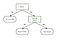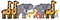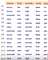# Decision Tree Mathematics

Decision Tree is a tree shaped algorithm used to determine a course of action. Each branch of the tree represents a possible decision, occurrence or reaction.

## Information Theory:

Information Theory is the fundamentals of decision trees. In order for us to understand Decision Tree algorithm, we need to understand Information Theory.

The basic idea of information theory is that the “informational value” of a data-set depends on the degree to which the content of the message is surprising or messy. If an event is very probable, it is no surprise (and generally uninteresting) when that event happens as expected; hence transmission of such a message carries very little new information. However, if an event is unlikely to occur, it is much more informative to learn that the event happened or will happen.

For example, there are at-least 3000 varieties of fishes available in both coast of India alone, if we are building a “shark” classifier to identify whether, it is a Shark or not. It is important for us to introduce non-shark images to the datasets as negative samples in-order for the classifier to distinguish between a shark and a non-shark fish. The entropy is high only when the mixture of positive samples (shark) and negative samples (non-shark) are equal, implying the data-set has interesting features to learn.

If all images in our data-set are positive(shark), then we don’t learn anything new — no matter how many images of shark it contains.

It is observed that the entropy is maximum when the probabilities are equal.

## Decision Tree:

The basic idea behind a Decision Tree is to break classification down into a set of choices about each entry (i.e. column) in our feature vector. We start at the root of the tree and then progress down to the leaves where the actual classification is made.

For example, lets assume we are on a hiking trip to lake district and the rain is on and off, we need to decide whether to stay indoor or go on a hike.FIG 1: AN EXAMPLE OF A DECISION TREE THAT WE CAN USE TO DECIDE IF WE WANT TO GO ON A HIKE OR STAY INDOOR

As you can see from FIG 1 above, we have created a decision tree diagram where the decision blocks (rectangles) indicate a choice that we must make. We then have branches which lead us to other decision blocks or a terminating block (ovals). A terminating block is a leaf node of the tree, indicating that a final decision has been made.

## Tree Construction:

Decision tree algorithms use information theory in some shape or form to obtain the optimal, most informative splits (i.e. the “decisions”) to construct a series of “if/then” rules in a tree-like manner.

First step in constructing a decision tree is forming the root node of the tree. ie, which feature is split to form the rest of the tree.

In order for us to find the root node, we calculate the information gain from each feature column. The feature column with maximum information gain is selected as the root node and split is made from the selected root node to construct the decision tree.

Formula to calculate Entropy :

Where , p = number of unique values in a feature column / total number of features in a feature column

For example, Lets find the entropy of the below animal datasetFIG 2 : ANIMAL DATASET

The dataset is looking quite messy and the entropy is high in this case

Total number of animals= 8

Number of Giraffe in Dataset = 3

Number of Tiger in Dataset = 2

Number of Monkey in Dataset = 1

Number of Elephant in Dataset = 2

Hence the entropy is calculated as below,

`import mathentropy = -(3/8)*math.log2(3/8)+(2/8)*math.log2(2/8)+(1/8)*math.log2(1/8)+(2/8)*math.log2(2/8)print(entropy)`

The entropy here is approximately 1.9. This is considered a high entropy , a high level of disorder ( meaning low level of purity).

# Example : Decision Tree

Lets take an example data-set below and build a decision tree on it. Here “play” feature is the independent variable and rest of the feature columns are dependent variables.FIG 3: SAMPLE DATASET TO DECIDE WHETHER TO PLAY OR NOT PLAY

We will take 5 steps to build the decision tree:

• Step 1: Compute the Entropy of data-set (target variable) — E(s)
• Step 2: Compute Information gain for each dependent variable — Gain(Outlook), Gain(temp), Gain(humidity), Gain(windy) using the below equations:

I(outlook) = ((total number of sunny /total features in outlook) * E(outlook=sunny)) * ((total number of overcast/total features in outlook) E(outlook=overcast)) * ((total number of rainy/total features in outlook) E(outlook=rainy))

Gain(outlook) = E(s) — I(outlook)

I(temp) = ((total number of hot/total features in temp) * E(temp=hot)) * ((total number of mild/total features in temp) E(temp=mild)) * ((total number of cool/total features in temp) E(temp=cool))

Gain(temp) = E(s) — I(temp)

I(humidity) = ((total number of high/total features in humidity) * E(humidity=high)) * ((total number of normal/total features in humidity) E(humidity=normal))

Gain(humidity) = E(s) — I(humidity)

I(windy) = ((total number of true/total features in windy) * E(windy=true)) * ((total number of false/total features in windy) E(windy=false))

Gain(windy) = E(s) — I(windy)

• Step 3: Find the feature with maximum Gain and select that as root node Gain(Outlook) or Gain(temp) or Gain(humidity) or Gain(windy)
• Step 4: Find the next node to split the tree further
• Step 5: A decision tree would repeat this process as it grows deeper and deeper till either it reaches a pre-defined depth or no additional split can result in a higher information gain beyond a certain threshold which can also usually be specified as a hyper-parameter!

Step 1: Compute the Entropy of data-set (target variable) — E(s)

The entropy of data-set E(s) is 0.94

Step 2: Compute Information gain of each features

Feature 1: outlook

The information gain from the ‘outlook’ feature is 0.24

Feature 2: temp

Feature 3: Humidity

Feature 4: windy

Step 3: Find the feature with maximum Gain and select that as root node Gain(Outlook) or Gain(temp) or Gain(humidity) or Gain(windy)

So, the maximum gain is identified as 0.24 , hence Outlook is our ROOT Node

Step 4: With more than two features the first split is made on the most informative feature and then at every split the information gain for each additional feature needs to be recomputed because it would not be the same as the information gain from each feature by itself. The entropy and information gain would have to be calculated after one or more splits have already been made which would change the results.

Step 5: A decision tree would repeat this process as it grows deeper and deeper till either it reaches a pre-defined depth or no additional split can result in a higher information gain beyond a certain threshold which can also usually be specified as a hyper-parameter!

Hope you had a great insight on decision tree algorithm by understanding the computations behind information gain and entropy.

Do let me know your feedback in the comments

Bay of Tech : ”Affordable technology solutions to everyone” |BoT provides solutions in Industry 4.0 | This space is the perspective page of BoT

## More from BoT

Bay of Tech : ”Affordable technology solutions to everyone” |BoT provides solutions in Industry 4.0 | This space is the perspective page of BoT